Home > A2C > Chapter 7 > Lesson 7.1.5 > Problem7-74

7-74.
1. Simplify each expression in parts (a) through (c) below. Then complete part (d). Homework Help ✎

1.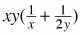2.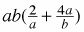)

3.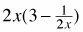4. What expression would go in the box to make the equation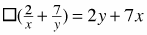true?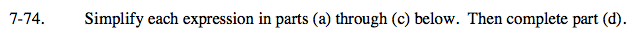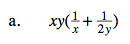Distribute the xy to both terms in the parentheses ( ).
Then simplify.

$\textit{y}+\frac{\textit{x}}{2}$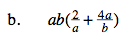2b + 4a2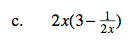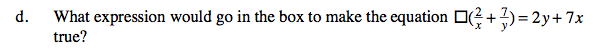See the previous parts of this problem for clues.